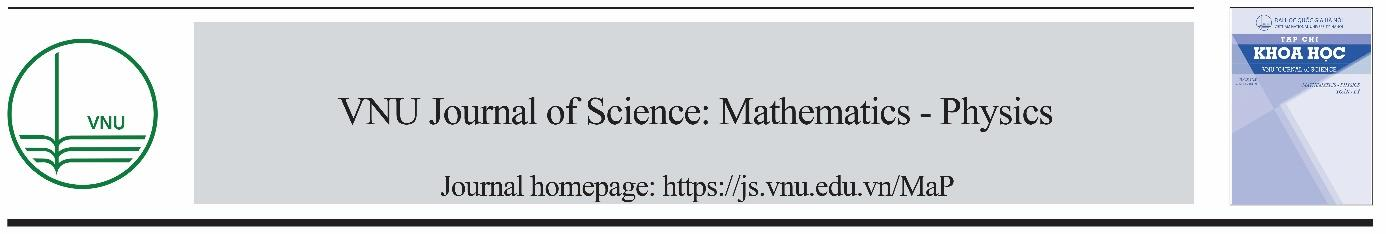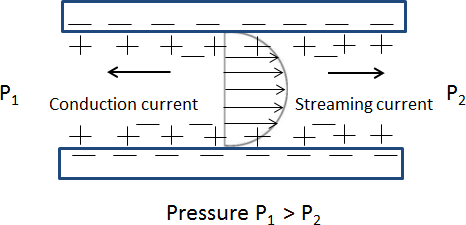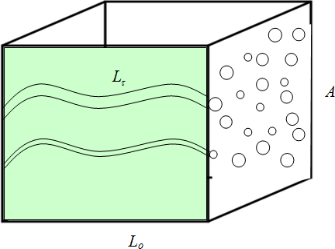# Examination of the fractal model for streaming potential coefficient in porous media

Đăng ngày 4/25/2019 3:45:33 AM | Thể loại: | Lần tải: 0 | Lần xem: 15 | Page: 12 | FileSize: 0.78 M | File type: PDF
Examination of the fractal model for streaming potential coefficient in porous media. In this work, the fractal model for the streaming potential coefficient in porous media recently published has been examined by calculating the zeta potential from the measured streaming potential coefficient. Obtained values of the zeta potential are then compared with experimental data.VNU Journal of Science: Mathematics Physics, Vol. 35, No. 1 (2019) 29-40
Original article
Examination of the Fractal Model for Streaming Potential
Coefficient in Porous Media
Luong Duy Thanh*
Thuy Loi University, 175 Tay Son, Dong Da, Hanoi, Vietnam
Received 26 September 2018
Revised 26 October 2018; Accepted 17 December 2018
Abstract: In this work, the fractal model for the streaming potential coefficient in porous media
recently published has been examined bycalculating the zeta potential from the measured streaming
potential coefficient. Obtained values of the zeta potential are then compared with experimental
data.
the
variation of the
streaming potential coefficient
with fluid
electrical
conductivity is predicted from the model. The results show that the model predictions are in good
agreement with the experimental data available in literature. The comparison between the proposed
model and the Helmholtz-Smoluchowski (HS) equation is also carried out. It is seen that the
prediction from the proposed model is quite close to what is expected from the HS equation, in
particularly at the high fluid conductivity or large grain diameters. Therefore, the model can be an
alternative approach to obtain the zeta potential from the streaming potential measurements.
Keywords: Streaming potential, zeta potential, fractal, porous media.
1. Introduction
Streaming potential measurements play an important role in geophysical applications. For example,
the streaming potential coefficient for various rock samples is one of the important factors in the
evaluation of seismoelectric well logging [e.g., 1, 2]. The streaming potential coefficient is also an
important
parameter
in
numerical
simulations
of
seismoelectric
exploration
[e.g.,
3,
4]
and
seismoelectric well logging [e.g., 5]. Streaming potential could be used to map subsurface flow and
detectsubsurfaceflowpatternsinoilreservoirs [e.g., 6, 7], geothermal areas and volcanoes [e.g., 8, 9],
detection of contaminant plumes [e.g., 10, 11]. It has also been proposed to use the streaming potential
________
Corresponding author.
https//doi.org/ 10.25073/2588-1124/vnumap.4306
2930
L.D. Thanh / VNU Journal of Science: Mathematics Physics, Vol. 35, No. 1 (2019) 29-40
monitoring to detect at distance the propagation of a water front in a reservoir [e.g., 12]. Monitoring of
streaming potential anomalies has been proposed as a means of predicting earthquakes [e.g., 13, 14].
Fractal models on porous media have attracted increasing interests from many different disciplines
[e.g., 15-22]. Recently, Luong et al.  have presented a fractal model for the streaming potential
coefficient in porous media based on the fractal theory of porous media and on the streaming potential
in a capillary. The proposed model has been applied to explain the dependence of the streaming
potential coefficient on the grain size.
The prediction is then compared with experimental data
available in the literature and good agreement is found between them. However, the model is not yet
examined more extensively.
In this work, the fractal model for the streaming potential coefficient in porous media presented in
 is examined by calculating the zeta potential that is normally determined by a conventional
Helmholtz- Smoluchowski (HS) equation. Obtained values are then compared with experimental data
available in literature. The result shows that the predicted zeta potential is in good agreement with the
experimental data. The comparison between the proposed model and the HS equation is also carried
out by plotting the ratio of the SPC as a function of particle diameter. It is shown that that the proposed
model is able to reproduce the similar result to the HS equation, in particularly at the high fluid
conductivity or largegrain diameters.
Figure 1. Development of streaming potential when an electrolyte is pumped through a capillary
(a porous medium is made of an array of capillaries).
2. Theoretical background
When a porous medium is saturated with an electrolyte, an electric double layer is formed on the
interface between the solid and the fluid. Some ions are absorbed into the solid surface and other ions
remain movable in the fluid. When a pressure difference is applied across a fluid saturated porous
medium, the relative motion happens between the pore fluid and solid grain surface. Then the net ions
of the diffuse layers move along with the flowing fluid at the same time. This movement of the net ions
generates a convection current (called streaming current) in the capillaries (a porous medium can be
approximated as an array of capillaries). The movement of the ions in the diffuse layer also makes the
separation of the positive and negative ions. Thus, an electric potential (streaming potential) is created
and that induces a conduction current in opposite direction to the streaming current as shown in Fig.
1). The streaming potential coefficient (SPC) is a key parameter that relates the pressure difference
(∆P) and the streaming potential difference (∆V) when the total current density (j) is zero asΔV
C =
,
Λ
d
C =
= .
Λ
d
L.D. Thanh / VNU Journal of Science: Mathematics Physics, Vol. 35, No. 1 (2019) 29-40
31
CS = ΔP j=0
(1)
The streaming potential coefficient in porous media is given by [e.g., 25, 26]
ro
S eff
(2)
where εr is the relative permittivity of the fluid, εo is the dielectric permittivity in vacuum, η is the
dynamic viscosity of the fluid, σeff is the effective conductivity, and ζ is the zeta potential which is the
electrical potential associated with the counter charge in the electrical double layer at the mineral-fluid
interface. The effective conductivity including the bulk fluid conductivity and the surface conductivity
is given by [e.g., 25, 27, 28]
eff = Kb + 2Ks ,
(3)
where Kb is the bulk fluid conductivity, Ks the specific surface conductance, Λ is a characteristic
length scale that describes the size of the pore network. There have been several models that relate
the characteristic length scale to grain diameter. One is given by 
Λ = 2m(F 1) ,
(4)
where d is the mean grain diameter, F is the formation factor (no units), m is the cementation
exponent of porous media (no units).
Consequently,
Eq.
(2)
ro ro
S (Kb + 2Ks ) (Kb + 2mKs (F 1))
can be rewritten as
(5)
Eq. (2) and therefore, Eq. (5) are known as the modified HS equation as mentioned above.
Figure 2. A porous medium composed of a large number of tortuous capillaries with random radius.

Bước 1:Tại trang tài liệu slideshare.vn bạn muốn tải, click vào nút Download màu xanh lá cây ở phía trên.
Bước 2: Tại liên kết tải về, bạn chọn liên kết để tải File về máy tính. Tại đây sẽ có lựa chọn tải File được lưu trên slideshare.vn
Bước 3: Một thông báo xuất hiện ở phía cuối trình duyệt, hỏi bạn muốn lưu . - Nếu click vào Save, file sẽ được lưu về máy (Quá trình tải file nhanh hay chậm phụ thuộc vào đường truyền internet, dung lượng file bạn muốn tải)
Có nhiều phần mềm hỗ trợ việc download file về máy tính với tốc độ tải file nhanh như: Internet Download Manager (IDM), Free Download Manager, ... Tùy vào sở thích của từng người mà người dùng chọn lựa phần mềm hỗ trợ download cho máy tính của mình
15 lần xem

## Tài liệu liên quan#### Examination of the fractal model for streaming potential coefficient in porous media#### The Temperature Dependence of the Zeta Potential in Porous Media#### A study on the Variation of Streaming Potential Coefficient with Physical Parameters of Rocks#### Numerical model of three phase three dimensional flow in porous media for reservoir simulation#### Báo cáo DETERMINING FLOW ENERGY AND EROSION COEFFICIENT FOR CLASSIFICATION OF POTENTIAL EROSION IN BINH DINH PROVINCE#### Calculation of the Ettingshausen Coefficient in a Rectangular Quantum Wire with an Infinite Potential in the Presence of an Electromagnetic Wave#### Replication Strategies for Streaming Media#### Model Policy Guidelines for the Appropriate Use of Social Media and Social Networking in Medical Practice#### Heat & Mass Transfer in Porous Media#### POSITIONING OF MEDIA ECONOMIC FRAMEWORK IN THE FIELD OF PROJECT BUSINESS FOR NOVEL MEDIA#### Streaming Potential in Unconsolidated Samples Saturated with Monovalent Electrolytes#### On a programme for the balancing calculation of flexible rotors with the influence coefficient method#### Báo cáo lâm nghiệp: A fractal root model applied for estimating the root biomass and architecture in two tropical legume tree species#### Pore size distribution in simulation of mass transport in porous media: A case study in reservoir analysis#### STOCHASTIC DYNAMICS Modeling Solute Transport in Porous Media#### Báo cáo y học: A quantitative evaluation of gross versus histologic neuroma formation in a rabbit forelimb amputation model: potential implications for the operative treatment and study of neuromas#### Computational Modelling of Multi-Scale Non-Fickian Dispersion in Porous Media - An Approach Based on Stochastic Calculus#### FAQs Tuberculosis Component of the Technical Instructions (TIs) for the Medical Examination of Aliens in the United States#### Heat and Mass Transfer in Porous Media